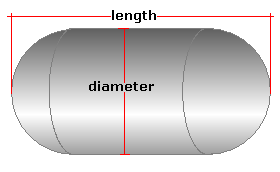Spike's Calculators

# Horizontal Cylindrical Tank with Hemispherical Heads - FeetCalculate the volume, capacity, and surface area of a horizontal cylindrical tank with hemispherical heads using feet for measurements. The shape of the tank looks like a capsule.
This calculator uses feet for measurements.

##### Approximate weight of Fuel or Water
```one US gallon of water = 8.34 pounds.
one US gallon of diesel fuel = 7.1 pounds
one US gallon of gas = 6 pounds
```
`Note: measurement used in the calculation is the  diameter, not the radius ! The length measurement is the overall tank length, cylinder plus the two caps.`

### Cylinder Tank Horizontal

Tank Diameter ft in
Length of the Tank ft in
Liquid Weight-Pounds per US Gallon lb/gal US

#### Results:

###### Tank Volume:
In Cubic Feet ft³
In Cubic Inches in³
In Cubic Metres
###### Tank Capacity:
In US Gallons gal US
In Imperial Gallons gal Imperial
In Litres L
###### Liquid Weight and Surface Area
Weight of Liquid lbs
Surface Area in Square Inches in²
In Square Feet ft²
In Square Millimetres mm²
In Square Metres

#### Calculator

1. he diameter of the horizontal cylinder tank in feet
2. the length of the tank in feet
3. the weight of the liquid in pounds per US gallon

#### Results

1. the volume of the tank in cubic feet
2. the volume in cubic inches
3. the volume in cubic metres
4. the tank capacity in US gallons
5. the capacity in Imperial gallons
6. the capacity in litres
7. full tank liquid weight based on lbs/gal US
8. the surface area of the tank in square inches
9. the surface area in square feet
10. the surface area in square millimetres
11. the surface area in square metres

##### Formula
```V=𝝅(D/2)²L
where V is the volume
𝝅 = 3.14159265
D the diameter
L the length of the cylinder part of the tank
```
##### Conversions
```one cubic foot (ft³) = 1728 cubic inches (in³)
one cubic foot (ft³) = 28316846.592 cubic millimetres (mm³)
one cubic foot (ft³) = 0.028316846592 cubic metres (m³)
one cubic foot (ft³) = 7.48051948051947 gallons (gal) US
one cubic foot (ft³) = 6.22883545904283 gallons (gal) Imperial
one square foot (ft²) = 144 square inches (in²)
one square foot (ft²) = 92903.04 square millimetres (mm²)
one square foot (ft²) = 0.09290304 square metres (m²)```

### Horizontal Cylindrical Tank with Hemispherical Heads (Capsule)#### Filled